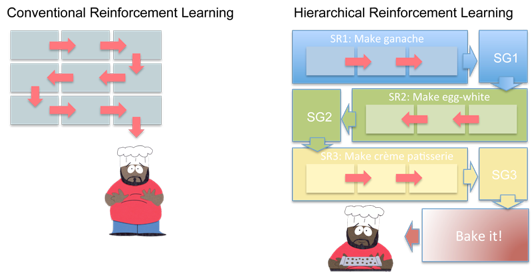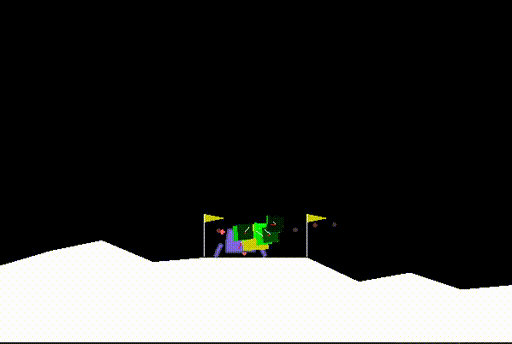# Goals as explanations of reinforcement learning agentsAn agent solving the mountain car environment. The red square represents the current goal (the position it wants to reach and the speed it wants to have there). The green squares represent the future goals after that, and allow to have longer term insights into what the agent will do.

Deep learning had a great impact on reinforcement learning because it allowed us to solve problems that no previous technique could deal with, such as learning to play Go from scratch or beating professionals at Starcraft II.

Unfortunately, using deep learning makes the agent a sort of black box. We don't know exactly what it has learned, if it has learned the right thing and if it's picking actions for the right reasons.

## Hierarchical reinforcement learning

When you decide to buy a birthday gift for a friend, you decompose that problem into many subtasks: list what your friend likes, search for a thing that matches these preferences, find the best place to buy it, go there to buy it and finally offer the gift to your friend.Instead of considering simple actions, we define mega-actions or subgoals such as "Make ganache", "Make egg-white" and "Make crème-patissiere", which require many simple actions (Source).

Solving a problem by decomposing it into a series of goals and then solving each goal one by one is so natural to us that we don't even notice we're doing it. We even often decompose a goal into subgoals and a subgoal into sub-subgoals without a thought. So if this strategy works for us, couldn't it be useful for RL agents too?

That insight leads to Hierarchical Reinforcement learning. In Hierarchical RL, instead of learning a single policy, there is a hierarchy of policies. For example, the hierarchy could have 3 levels of policies to win at Starcraft:

1. A high level policy that chooses the current goal: defend from an attack, focus on economy or launch an aggressive attack against the enemy
2. A medium level policy that picks the most appropriate subgoal to accomplish the current goal: move this group of units or build that series of buildings
3. A low level policy that picks actions to complete the subgoal as fast and well as possible: move this soldier or build a house there

Why are all of these called policies, if the high and medium-level policies output a goal instead of an action? Because we consider goals as high level actions or mega-actions (composed of many simpler sub-actions).

The number of levels in the hierarchy is currently an arbitrary choice made by people, but an interesting idea would be to dynamically decompose goals into subgoals as the need arises instead of having a fixed amount of levels.

Of course, we don't want to manually As in the experiments for the Arcade Learning environment in , where they manually defined reaching a goal as being near a specific object say which goals and subgoals the agent should choose from, because we would need to define them for every new environment and the ones we manually picked could be suboptimal. It's better if the agent learns during the training process which goals to pick. It could also lead to finding approaches humans wouldn't have considered or thought about.

## What is a goal?

There are multiple ways we could define goal and each definition has specific consequences:

1. A discrete set of numbers, from 0 to X, and the sub-agent would learn to associate behaviors with each number. For example, 0 could mean "build a house" and 1 "attack the enemy". The disadvantage is that we need to pick in advance how many goals there are and it's not very clear what a number corresponds to (if we see that agent picked goal 4, what is it actually trying to do?)

2. A vector of N dimensions . We don't have to decide anymore how many goals exists (there is an infinity) but we still have the problem that a goal isn't directly understandable by a human (what does $[0.13, 4.1, -2.7]$) mean?).

3. A desired end state (e.g. "I want to observe this" As in the first experiments in , where each of the 6 possible states is a goal ). This is already much better for interpretability, but has some problems of generalization. Imagine we want to teach an agent to walk in a straight line. We would need to specify many points along the way as subgoals and would lose the obvious generality of "walk straight". Additionally, if there's a good state the agent has never seen, it could be difficult for the agent to learn to pick it as a goal.

4. A direction in the state space. This would be more general than a specific state, because the goal now represents a constant change we want:

$s_{desired} = s_{current} + g$

We don't actually know in advance the desired state $s_{desired}$ but hope the agent learns to pick goals / directions that lead to moving towards a good end state.

This definition suffers from one weakness: if the environment is stochastic, we might end up in an unexpected state and thus it would be better to adjust the direction. For example, imagine a maze environment where the floor is slippery, so the agent might randomly slip to a neighboring place. If the maze exit is north, the goal might be "go north". However, if the agent slips in one of the steps, going north could now lead to hitting a wall and getting stuck, and so going north wouldn't be the correct goal anymore. In this sense, a fixed direction isn't flexible enough: we should adapt it as we do steps to ensure we're still moving to the right state.

5. A change in state. This is very similar to a direction, except that at each step we notice the new state we're in and adjust the goal accordingly. We want $s_{desired} = s + g = s' + g'$ and therefore $g' = s + g - s'$, where $s$ is the previous state, $s'$ is the new state and $g$ and $g'$ are the previous goal and the new goal, respectively.

Nachum et al. used this last definition to great effect in a paper where they also added off-policy training and use of a experience replay buffer, which lead to more sample-efficient learning.

## Learning the goals

How are these goals learned? And how do we know that the learned goals actually correspond to the definition above and aren't just a big vector with direct meaning?

Different papers take different approaches. Kulkarni et al (2016, ) learned a set of relevant objects in each Atari game and then use "go near that object" as a goal. Therefore, this corresponded to a fairly small number of goals and was easily learned. The high-level policy had to pick among the pre-defined goals (it was therefore similar to definition 1, except that we hand picked the goal).

Vezhnevets et al (2017, ) picked the N-dimensional vector as the definition of a goal. The high-level policy used a neural network to pick that vector. At the beginning, when the network had learned nothing, the goal was almost random. The low-level policy then had to pick actions according to that goal, which at the beginning was also random. However, as training progresses, the low-level policy sometimes picked better actions by chance and the high-level policy learned to associate high rewards with the goal that was used at that time. This bootstrapped the process and over time the high-level learned to pick vector that made the low-level policy act well and the low-level policy picked actions that led to reaching the goal as fast and well as possible (this was the way the low-level policy loss was defined).

In Nachum et al (2018, ) the goal is seen as a desired change in state (Definition 5). At each step the desired changed is adjusted and the low-level policy picks in order to make the desired change as small as possible (because it means we're very close to the goal). The high-level policy works in the same way as the previous paper. Both policies use DDPG and to improve the sample efficiency, they use an experience replay buffer (with some adjustemts due to using Hierarchical RL).

## Explainable agents

Once we have this hierarchy of policies, we can understand the agent much better, since the goals allow us to understand the states the agent is trying to reach:

$s_{desired} = s_{current} + g$

If there are multiple hierarchies, we can visualise the goals of the agent in many time scales (short term, medium term, long term, ...).

Seeing the desired states is useful but another key component of explainability is understanding why those goals were chosen. To do so, we can use more traditional techniques that examine the link between the state and the chosen action / goal:

• Saliency and attribution: how important was each part of the input to pick the current goal / action?
• Importance maps: force the agent to focus on a subset of the state to see which one is the most important.
• Policy distillation: by decomposing the problem, each agent might be simple enough to be distilled into a interpretable model with good performance.

## It's easier to understand the decisions of the agent if we know the agent's goals

The key idea is simple: if we know the series of goals the agent is trying to reach, its actions become much clearer and we can predict what it will do in the future. I developer 3 key contributions to do so in my Master Thesis:

1. Create goal explanations: we can understand the agent better if we know its goals
2. Discover a process to obtain those goals: use hierarchical agents
3. Develop new algorithms to train hierarchical agents. These algorithms are better than the state-of-the-art, making it possible to train hierarchical agents in more difficult environments such as Lunar Lander.

You can observe the resulting explanations in the examples below:An agent solving the mountain car environment. The red square represents the current goal (the position it wants to reach and the speed it wants to have there). The green squares represent the future goals after that, showing us the agent's plan to reach the top of the mountain on the right.An agent solving the mountain car environment. The red yellow represents the current goal (the position it wants to reach, as well as the speed, angle, and angular momentum it wants) The green squares represent the future goals after the current goal, and allow us to know the agent's plan to solve the task and reach the ground safely.